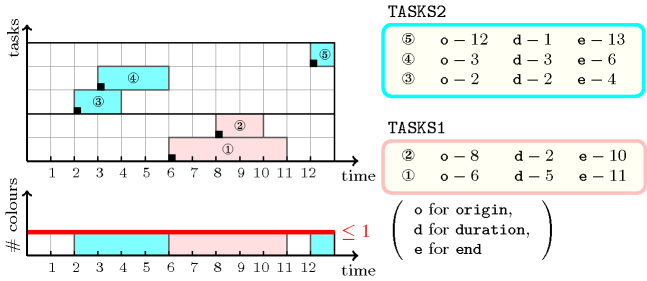Origin
Constraint

$\mathrm{𝚍𝚒𝚜𝚓𝚘𝚒𝚗𝚝}_\mathrm{𝚝𝚊𝚜𝚔𝚜}\left(\mathrm{𝚃𝙰𝚂𝙺𝚂}\mathtt{1},\mathrm{𝚃𝙰𝚂𝙺𝚂}\mathtt{2}\right)$

Arguments
 $\mathrm{𝚃𝙰𝚂𝙺𝚂}\mathtt{1}$ $\mathrm{𝚌𝚘𝚕𝚕𝚎𝚌𝚝𝚒𝚘𝚗}\left(\mathrm{𝚘𝚛𝚒𝚐𝚒𝚗}-\mathrm{𝚍𝚟𝚊𝚛},\mathrm{𝚍𝚞𝚛𝚊𝚝𝚒𝚘𝚗}-\mathrm{𝚍𝚟𝚊𝚛},\mathrm{𝚎𝚗𝚍}-\mathrm{𝚍𝚟𝚊𝚛}\right)$ $\mathrm{𝚃𝙰𝚂𝙺𝚂}\mathtt{2}$ $\mathrm{𝚌𝚘𝚕𝚕𝚎𝚌𝚝𝚒𝚘𝚗}\left(\mathrm{𝚘𝚛𝚒𝚐𝚒𝚗}-\mathrm{𝚍𝚟𝚊𝚛},\mathrm{𝚍𝚞𝚛𝚊𝚝𝚒𝚘𝚗}-\mathrm{𝚍𝚟𝚊𝚛},\mathrm{𝚎𝚗𝚍}-\mathrm{𝚍𝚟𝚊𝚛}\right)$
Restrictions
 $\mathrm{𝚛𝚎𝚚𝚞𝚒𝚛𝚎}_\mathrm{𝚊𝚝}_\mathrm{𝚕𝚎𝚊𝚜𝚝}$$\left(2,\mathrm{𝚃𝙰𝚂𝙺𝚂}\mathtt{1},\left[\mathrm{𝚘𝚛𝚒𝚐𝚒𝚗},\mathrm{𝚍𝚞𝚛𝚊𝚝𝚒𝚘𝚗},\mathrm{𝚎𝚗𝚍}\right]\right)$ $\mathrm{𝚃𝙰𝚂𝙺𝚂}\mathtt{1}.\mathrm{𝚍𝚞𝚛𝚊𝚝𝚒𝚘𝚗}\ge 0$ $\mathrm{𝚃𝙰𝚂𝙺𝚂}\mathtt{1}.\mathrm{𝚘𝚛𝚒𝚐𝚒𝚗}\le \mathrm{𝚃𝙰𝚂𝙺𝚂}\mathtt{1}.\mathrm{𝚎𝚗𝚍}$ $\mathrm{𝚛𝚎𝚚𝚞𝚒𝚛𝚎}_\mathrm{𝚊𝚝}_\mathrm{𝚕𝚎𝚊𝚜𝚝}$$\left(2,\mathrm{𝚃𝙰𝚂𝙺𝚂}\mathtt{2},\left[\mathrm{𝚘𝚛𝚒𝚐𝚒𝚗},\mathrm{𝚍𝚞𝚛𝚊𝚝𝚒𝚘𝚗},\mathrm{𝚎𝚗𝚍}\right]\right)$ $\mathrm{𝚃𝙰𝚂𝙺𝚂}\mathtt{2}.\mathrm{𝚍𝚞𝚛𝚊𝚝𝚒𝚘𝚗}\ge 0$ $\mathrm{𝚃𝙰𝚂𝙺𝚂}\mathtt{2}.\mathrm{𝚘𝚛𝚒𝚐𝚒𝚗}\le \mathrm{𝚃𝙰𝚂𝙺𝚂}\mathtt{2}.\mathrm{𝚎𝚗𝚍}$
Purpose

Each task of the collection $\mathrm{𝚃𝙰𝚂𝙺𝚂}\mathtt{1}$ should not overlap any task of the collection $\mathrm{𝚃𝙰𝚂𝙺𝚂}\mathtt{2}$. Two tasks overlap if they have an intersection that is strictly greater than zero.

Example
$\left(\begin{array}{c}〈\begin{array}{ccc}\mathrm{𝚘𝚛𝚒𝚐𝚒𝚗}-6\hfill & \mathrm{𝚍𝚞𝚛𝚊𝚝𝚒𝚘𝚗}-5\hfill & \mathrm{𝚎𝚗𝚍}-11,\hfill \\ \mathrm{𝚘𝚛𝚒𝚐𝚒𝚗}-8\hfill & \mathrm{𝚍𝚞𝚛𝚊𝚝𝚒𝚘𝚗}-2\hfill & \mathrm{𝚎𝚗𝚍}-10\hfill \end{array}〉,\hfill \\ 〈\begin{array}{ccc}\mathrm{𝚘𝚛𝚒𝚐𝚒𝚗}-2\hfill & \mathrm{𝚍𝚞𝚛𝚊𝚝𝚒𝚘𝚗}-2\hfill & \mathrm{𝚎𝚗𝚍}-4,\hfill \\ \mathrm{𝚘𝚛𝚒𝚐𝚒𝚗}-3\hfill & \mathrm{𝚍𝚞𝚛𝚊𝚝𝚒𝚘𝚗}-3\hfill & \mathrm{𝚎𝚗𝚍}-6,\hfill \\ \mathrm{𝚘𝚛𝚒𝚐𝚒𝚗}-12\hfill & \mathrm{𝚍𝚞𝚛𝚊𝚝𝚒𝚘𝚗}-1\hfill & \mathrm{𝚎𝚗𝚍}-13\hfill \end{array}〉\hfill \end{array}\right)$

Figure 5.125.1 displays the two groups of tasks (i.e., the tasks of $\mathrm{𝚃𝙰𝚂𝙺𝚂}\mathtt{1}$ and the tasks of $\mathrm{𝚃𝙰𝚂𝙺𝚂}\mathtt{2}$). Since no task of the first group overlaps any task of the second group, the $\mathrm{𝚍𝚒𝚜𝚓𝚘𝚒𝚗𝚝}_\mathrm{𝚝𝚊𝚜𝚔𝚜}$ constraint holds.

##### Figure 5.125.1. The $\mathrm{𝚍𝚒𝚜𝚓𝚘𝚒𝚗𝚝}_\mathrm{𝚝𝚊𝚜𝚔𝚜}$ solution to the Example slot with at most one distinct colour in parallel (tasks in $\mathrm{𝚃𝙰𝚂𝙺𝚂}\mathtt{1}$ have the pink colour, while tasks in $\mathrm{𝚃𝙰𝚂𝙺𝚂}\mathtt{2}$ have the blue colour)Typical
 $|\mathrm{𝚃𝙰𝚂𝙺𝚂}\mathtt{1}|>1$ $\mathrm{𝚃𝙰𝚂𝙺𝚂}\mathtt{1}.\mathrm{𝚍𝚞𝚛𝚊𝚝𝚒𝚘𝚗}>0$ $|\mathrm{𝚃𝙰𝚂𝙺𝚂}\mathtt{2}|>1$ $\mathrm{𝚃𝙰𝚂𝙺𝚂}\mathtt{2}.\mathrm{𝚍𝚞𝚛𝚊𝚝𝚒𝚘𝚗}>0$
Symmetries
• Arguments are permutable w.r.t. permutation $\left(\mathrm{𝚃𝙰𝚂𝙺𝚂}\mathtt{1},\mathrm{𝚃𝙰𝚂𝙺𝚂}\mathtt{2}\right)$.

• Items of $\mathrm{𝚃𝙰𝚂𝙺𝚂}\mathtt{1}$ are permutable.

• Items of $\mathrm{𝚃𝙰𝚂𝙺𝚂}\mathtt{2}$ are permutable.

• One and the same constant can be added to the $\mathrm{𝚘𝚛𝚒𝚐𝚒𝚗}$ and $\mathrm{𝚎𝚗𝚍}$ attributes of all items of $\mathrm{𝚃𝙰𝚂𝙺𝚂}\mathtt{1}$ and $\mathrm{𝚃𝙰𝚂𝙺𝚂}\mathtt{2}$.

Arg. properties
• Contractible wrt. $\mathrm{𝚃𝙰𝚂𝙺𝚂}\mathtt{1}$.

• Contractible wrt. $\mathrm{𝚃𝙰𝚂𝙺𝚂}\mathtt{2}$.

Remark

Despite the fact that this is not an uncommon constraint, it cannot be modelled in a compact way with a single $\mathrm{𝚌𝚞𝚖𝚞𝚕𝚊𝚝𝚒𝚟𝚎}$ constraint. But it can be expressed by using the $\mathrm{𝚌𝚘𝚕𝚘𝚞𝚛𝚎𝚍}_\mathrm{𝚌𝚞𝚖𝚞𝚕𝚊𝚝𝚒𝚟𝚎}$ constraint: We assign a first colour to the tasks of $\mathrm{𝚃𝙰𝚂𝙺𝚂}\mathtt{1}$ as well as a second distinct colour to the tasks of $\mathrm{𝚃𝙰𝚂𝙺𝚂}\mathtt{2}$. Finally we set up a limit of 1 for the maximum number of distinct colours allowed at each time point.

Reformulation

The $\mathrm{𝚍𝚒𝚜𝚓𝚘𝚒𝚗𝚝}_\mathrm{𝚝𝚊𝚜𝚔𝚜}$ constraint can be expressed in term of $|\mathrm{𝚃𝙰𝚂𝙺𝚂}\mathtt{1}|·|\mathrm{𝚃𝙰𝚂𝙺𝚂}\mathtt{2}|$ reified constraints. For each task $\mathrm{𝚃𝙰𝚂𝙺𝚂}\mathtt{1}\left[i\right]$ $\left(i\in \left[1,|\mathrm{𝚃𝙰𝚂𝙺𝚂}\mathtt{1}|\right]\right)$ and for each task $\mathrm{𝚃𝙰𝚂𝙺𝚂}\mathtt{2}\left[j\right]$ $\left(j\in \left[1,|\mathrm{𝚃𝙰𝚂𝙺𝚂}\mathtt{2}|\right]\right)$ we generate a reified constraint of the form $\mathrm{𝚃𝙰𝚂𝙺𝚂}\mathtt{1}\left[i\right].\mathrm{𝚎𝚗𝚍}\le \mathrm{𝚃𝙰𝚂𝙺𝚂}\mathtt{2}\left[j\right].\mathrm{𝚘𝚛𝚒𝚐𝚒𝚗}\vee \mathrm{𝚃𝙰𝚂𝙺𝚂}\mathtt{2}\left[j\right].\mathrm{𝚎𝚗𝚍}\le \mathrm{𝚃𝙰𝚂𝙺𝚂}\mathtt{1}\left[i\right].\mathrm{𝚘𝚛𝚒𝚐𝚒𝚗}$. In addition we also state for each task an arithmetic constraint that states that the end of a task is equal to the sum of its origin and its duration.

Systems

generalisation: $\mathrm{𝚌𝚘𝚕𝚘𝚞𝚛𝚎𝚍}_\mathrm{𝚌𝚞𝚖𝚞𝚕𝚊𝚝𝚒𝚟𝚎}$ (tasks colours and limit on maximum number of colours in parallel are explicitly given).

specialisation: $\mathrm{𝚍𝚒𝚜𝚓𝚘𝚒𝚗𝚝}$ ($\mathrm{𝚝𝚊𝚜𝚔}$ replaced by $\mathrm{𝚟𝚊𝚛𝚒𝚊𝚋𝚕𝚎}$).

Keywords
Arc input(s)

$\mathrm{𝚃𝙰𝚂𝙺𝚂}\mathtt{1}$

Arc generator
$\mathrm{𝑆𝐸𝐿𝐹}$$↦\mathrm{𝚌𝚘𝚕𝚕𝚎𝚌𝚝𝚒𝚘𝚗}\left(\mathrm{𝚝𝚊𝚜𝚔𝚜}\mathtt{1}\right)$

Arc arity
Arc constraint(s)
$\mathrm{𝚝𝚊𝚜𝚔𝚜}\mathtt{1}.\mathrm{𝚘𝚛𝚒𝚐𝚒𝚗}+\mathrm{𝚝𝚊𝚜𝚔𝚜}\mathtt{1}.\mathrm{𝚍𝚞𝚛𝚊𝚝𝚒𝚘𝚗}=\mathrm{𝚝𝚊𝚜𝚔𝚜}\mathtt{1}.\mathrm{𝚎𝚗𝚍}$
Graph property(ies)
$\mathrm{𝐍𝐀𝐑𝐂}$$=|\mathrm{𝚃𝙰𝚂𝙺𝚂}\mathtt{1}|$

Arc input(s)

$\mathrm{𝚃𝙰𝚂𝙺𝚂}\mathtt{2}$

Arc generator
$\mathrm{𝑆𝐸𝐿𝐹}$$↦\mathrm{𝚌𝚘𝚕𝚕𝚎𝚌𝚝𝚒𝚘𝚗}\left(\mathrm{𝚝𝚊𝚜𝚔𝚜}\mathtt{2}\right)$

Arc arity
Arc constraint(s)
$\mathrm{𝚝𝚊𝚜𝚔𝚜}\mathtt{2}.\mathrm{𝚘𝚛𝚒𝚐𝚒𝚗}+\mathrm{𝚝𝚊𝚜𝚔𝚜}\mathtt{2}.\mathrm{𝚍𝚞𝚛𝚊𝚝𝚒𝚘𝚗}=\mathrm{𝚝𝚊𝚜𝚔𝚜}\mathtt{2}.\mathrm{𝚎𝚗𝚍}$
Graph property(ies)
$\mathrm{𝐍𝐀𝐑𝐂}$$=|\mathrm{𝚃𝙰𝚂𝙺𝚂}\mathtt{2}|$

Arc input(s)

$\mathrm{𝚃𝙰𝚂𝙺𝚂}\mathtt{1}$ $\mathrm{𝚃𝙰𝚂𝙺𝚂}\mathtt{2}$

Arc generator
$\mathrm{𝑃𝑅𝑂𝐷𝑈𝐶𝑇}$$↦\mathrm{𝚌𝚘𝚕𝚕𝚎𝚌𝚝𝚒𝚘𝚗}\left(\mathrm{𝚝𝚊𝚜𝚔𝚜}\mathtt{1},\mathrm{𝚝𝚊𝚜𝚔𝚜}\mathtt{2}\right)$

Arc arity
Arc constraint(s)
 $•\mathrm{𝚝𝚊𝚜𝚔𝚜}\mathtt{1}.\mathrm{𝚍𝚞𝚛𝚊𝚝𝚒𝚘𝚗}>0$ $•\mathrm{𝚝𝚊𝚜𝚔𝚜}\mathtt{2}.\mathrm{𝚍𝚞𝚛𝚊𝚝𝚒𝚘𝚗}>0$ $•\mathrm{𝚝𝚊𝚜𝚔𝚜}\mathtt{1}.\mathrm{𝚘𝚛𝚒𝚐𝚒𝚗}<\mathrm{𝚝𝚊𝚜𝚔𝚜}\mathtt{2}.\mathrm{𝚎𝚗𝚍}$ $•\mathrm{𝚝𝚊𝚜𝚔𝚜}\mathtt{2}.\mathrm{𝚘𝚛𝚒𝚐𝚒𝚗}<\mathrm{𝚝𝚊𝚜𝚔𝚜}\mathtt{1}.\mathrm{𝚎𝚗𝚍}$
Graph property(ies)
$\mathrm{𝐍𝐀𝐑𝐂}$$=0$

Graph model

$\mathrm{𝑃𝑅𝑂𝐷𝑈𝐶𝑇}$ is used in order to generate the arcs of the graph between all the tasks of the collection $\mathrm{𝚃𝙰𝚂𝙺𝚂}\mathtt{1}$ and all tasks of the collection $\mathrm{𝚃𝙰𝚂𝙺𝚂}\mathtt{2}$. The first two graph constraints respectively enforce for each task of $\mathrm{𝚃𝙰𝚂𝙺𝚂}\mathtt{1}$ and $\mathrm{𝚃𝙰𝚂𝙺𝚂}\mathtt{2}$ the fact that the end of a task is equal to the sum of its origin and its duration. The arc constraint of the third graph constraint depicts the fact that two tasks overlap. Therefore, since we use the graph property $\mathrm{𝐍𝐀𝐑𝐂}$ $=$ 0 the final graph associated with the third graph constraint will be empty and no task of $\mathrm{𝚃𝙰𝚂𝙺𝚂}\mathtt{1}$ will overlap any task of $\mathrm{𝚃𝙰𝚂𝙺𝚂}\mathtt{2}$. Figure 5.125.2 shows the initial graph of the third graph constraint associated with the Example slot. Because of the graph property $\mathrm{𝐍𝐀𝐑𝐂}$ $=$ 0 the corresponding final graph is empty.

##### Figure 5.125.2. Initial graph of the $\mathrm{𝚍𝚒𝚜𝚓𝚘𝚒𝚗𝚝}_\mathrm{𝚝𝚊𝚜𝚔𝚜}$ constraint (the final graph is empty)Signature

Since $\mathrm{𝚃𝙰𝚂𝙺𝚂}\mathtt{1}$ is the maximum number of arcs of the final graph associated with the first graph constraint we can rewrite $\mathrm{𝐍𝐀𝐑𝐂}$ $=$ $|\mathrm{𝚃𝙰𝚂𝙺𝚂}\mathtt{1}|$. This leads to simplify $\underline{\overline{\mathrm{𝐍𝐀𝐑𝐂}}}$ to $\overline{\mathrm{𝐍𝐀𝐑𝐂}}$.

We can apply a similar remark for the second graph constraint.

Finally, since 0 is the smallest number of arcs of the final graph we can rewrite $\mathrm{𝐍𝐀𝐑𝐂}$ $=$ 0 to $\mathrm{𝐍𝐀𝐑𝐂}$ $\le$ 0. This leads to simplify $\underline{\overline{\mathrm{𝐍𝐀𝐑𝐂}}}$ to $\underline{\mathrm{𝐍𝐀𝐑𝐂}}$.